Theory and Modern Applications

# Fixed points and quadratic equations connected with homomorphisms and derivations on non-Archimedean algebras

## Abstract

We apply the fixed point method to prove the stability of the systems of functional equations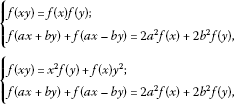on non-Archimedean Banach algebras. Moreover, we give some applications of our results in non-Archimedean Banach algebras over p-adic numbers.

MSC:34K36, 46S40, 47S40, 39B82, 39B52, 26E50.

## 1 Introduction and preliminaries

The story of the stability of functional equations dates back to 1925 when a stability result appeared in the celebrated book by Gy. Pólya and G. Szegő . In 1940, Ulam  posed the famous Ulam stability problem which was partially solved by Hyers  in the framework of Banach spaces. Later, Aoki  considered the stability problem with unbounded Cauchy differences. In 1978, Th. M. Rassias  provided a generalization of the Hyers’ theorem by proving the existence of unique linear mappings near approximate additive mappings. Gǎvruta  obtained a generalized result of Th. M. Rassias’ theorem which allows the Cauchy difference to be controlled by a general unbounded function. On the other hand, J. M. Rassias  considered the Cauchy difference controlled by a product of different powers of norm. However, there was a singular case; for this singularity, a counterexample was given by Gǎvruta .

Bourgin  proved the stability of ring homomorphisms in two unital Banach algebras. Badora  gave a generalization of the Bourgin’s result. The stability result concerning derivations on operator algebras was first obtained by Šemrl . In , Badora proved the stability of functional equation $f\left(xy\right)=xf\left(y\right)+f\left(x\right)y$, where f is a mapping on normed algebra A with unit.

Let $\mathcal{A}$, $\mathcal{B}$ be two algebras. A mapping $f:\mathcal{A}\to \mathcal{B}$ is called a quadratic homomorphism if f is a quadratic mapping satisfying $f\left(xy\right)=f\left(x\right)f\left(y\right)$ for all $x,y\in \mathcal{A}$. For instance, let $\mathcal{A}$ be commutative. Then the mapping $f:\mathcal{A}\to \mathcal{A}$, defined by $f\left(x\right)={x}^{2}$ ($x\in \mathcal{A}$), is a quadratic homomorphism.

A mapping $f:\mathcal{A}\to \mathcal{A}$ is called a quadratic derivation if f is a quadratic mapping satisfying $f\left(xy\right)={x}^{2}f\left(y\right)+f\left(x\right){y}^{2}$ for all $x,y\in \mathcal{A}$. For instance, consider the algebra of $2×2$ matrices

$\mathcal{A}=\left\{\left[\begin{array}{cc}{c}_{1}& {c}_{2}\\ 0& 0\end{array}\right]:{c}_{1},{c}_{2}\in \mathbb{C}\phantom{\rule{0.25em}{0ex}}\left(\text{complex field}\right)\right\}.$

Then it is easy to see that the mapping $f:\mathcal{A}\to \mathcal{A}$, defined by $f\left(\left[\begin{array}{cc}{c}_{1}& {c}_{2}\\ 0& 0\end{array}\right]\right)=\left[\begin{array}{cc}0& {c}_{2}^{2}\\ 0& 0\end{array}\right]$, is a quadratic derivation. We note that quadratic derivations and ring derivations are different.

Arriola and Beyer in  initiated the stability of functional equations in non-Archimedean spaces. In fact they established the stability of Cauchy functional equations over p-adic fields. After their results, some papers (see for instance ) on the stability of other equations in such spaces have been published. Although different methods are known for establishing the stability of functional equations, almost all proofs depend on the Hyers’s method in . In 2003, Radu  employed the alternative fixed point theorem, due to Diaz and Margolis , to prove the stability of the Cauchy additive functional equation. Subsequently, this method was applied to investigate the Hyers-Ulam stability for the Jensen functional equation , as well as for the Cauchy functional equation , by considering a general control function $\phi \left(x,y\right)$ with suitable properties. Using such an elegant idea, several authors applied the method to investigate the stability of some functional equations, see .

Recently, Eshaghi and Khodaei  considered the following quadratic functional equation:

$f\left(ax+by\right)+f\left(ax-by\right)=2{a}^{2}f\left(x\right)+2{b}^{2}f\left(y\right)$
(1.1)

and proved the Hyers-Ulam stability of the above functional equation in classical Banach spaces. Recently, Eshaghi  proved the Hyers-Ulam stability of homomorphisms and derivations on non-Archimedean Banach algebras.

In the present paper, we adopt the idea of Cǎdariu and Radu  to establish the stability of quadratic homomorphisms and quadratic derivations related to the quadratic functional equation (1.1) over non-Archimedean Banach algebras. Some applications of our results in non-Archimedean Banach algebras over p-adic numbers will be exhibited.

Now, we recall some notations and basic definitions used later on in the paper.

In 1897, Hensel  discovered the p-adic numbers as a number theoretical analogue of power series in complex analysis. During the last three decades p-adic numbers have gained the interest of physicists for their research, in particular in problems coming from quantum physics, p-adic strings and superstrings [37, 38]. A key property of p-adic numbers is that they do not satisfy the Archimedean axiom: For $x,y>0$, there exists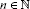such that $x (see [39, 40]).

Example 1.1 Let p be a prime number. For any nonzero rational number $a={p}^{r}\frac{m}{n}$ such that m and n are coprime to the prime number p, define the p-adic absolute value ${|a|}_{p}={p}^{-r}$. Then is a non-Archimedean norm on $\mathbb{Q}$. The completion of $\mathbb{Q}$ with respect to is denoted by ${\mathbb{Q}}_{p}$ and is called the p-adic number field. Note that if $p\ge 3$, then $|{2}^{n}|=1$ in for each integer n.

Let $\mathbb{K}$ denote a field and function (valuation absolute) $|\cdot |$ from $\mathbb{K}$ into $\left[0,\mathrm{\infty }\right)$. A non-Archimedean valuation is a function $|\cdot |$ that satisfies the strong triangle inequality; namely, $|x+y|\le max\left\{|x|,|y|\right\}\le |x|+|y|$ for all $x,y\in \mathbb{K}$. The associated field $\mathbb{K}$ is referred to as a non-Archimedean field. Clearly, $|1|=|-1|=1$ and $|n|\le 1$ for all $n\ge 1$. A trivial example of a non-Archimedean valuation is the function $|\cdot |$ taking everything except 0 into 1 and $|0|=0$. We always assume in addition that $|\cdot |$ is nontrivial, i.e., there is a $z\in \mathbb{K}$ such that $|z|\ne 0,1$.

Let X be a linear space over a field $\mathbb{K}$ with a non-Archimedean nontrivial valuation $|\cdot |$. A function $\parallel \cdot \parallel :X\to \left[0,\mathrm{\infty }\right)$ is said to be a non-Archimedean norm if it is a norm over $\mathbb{K}$ with the strong triangle inequality (ultrametric); namely, $\parallel x+y\parallel \le max\left\{\parallel x\parallel ,\parallel y\parallel \right\}$ for all $x,y\in X$. Then $\left(X,\parallel \cdot \parallel \right)$ is called a non-Archimedean space. In any such a space a sequence ${\left\{{x}_{n}\right\}}_{n\in \mathbb{N}}$ is Cauchy if and only if ${\left\{{x}_{n+1}-{x}_{n}\right\}}_{n\in \mathbb{N}}$ converges to zero. By a complete non-Archimedean space we mean one in which every Cauchy sequence is convergent. A non-Archimedean Banach algebra is a complete non-Archimedean algebra $\mathcal{A}$ which satisfies $\parallel xy\parallel \le \parallel x\parallel \parallel y\parallel$ for all $x,y\in \mathcal{A}$. For more details the reader is referred to [41, 42].

Let X be a nonempty set and $d:X×X\to \left[0,\mathrm{\infty }\right]$ satisfying: $d\left(x,y\right)=0$ if and only if $x=y$, $d\left(x,y\right)=d\left(y,x\right)$ and $d\left(x,z\right)\le max\left\{d\left(x,y\right),d\left(y,z\right)\right\}$ (strong triangle inequality), for all $x,y,z\in X$. Then $\left(X,d\right)$ is called a non-Archimedean generalized metric space. $\left(X,d\right)$ is called complete if every d-Cauchy sequence in X is d-convergent.

Using the strong triangle inequality in the proof of the main result of , we get to the following result:

Theorem 1.2 (Non-Archimedean Alternative Contraction Principle)

If $\left(\mathrm{\Omega },d\right)$ is a non-Archimedean generalized complete metric space and $T:\mathrm{\Omega }\to \mathrm{\Omega }$ a strictly contractive mapping (that is $d\left(T\left(x\right),T\left(y\right)\right)\le Ld\left(x,y\right)$, for all $x,y\in T$ and a Lipschitz constant $L<1$). Let $x\in \mathrm{\Omega }$, then either

1. (i)

$d\left({T}^{n}\left(x\right),{T}^{n+1}\left(x\right)\right)=\mathrm{\infty }$ for all $n\ge 0$, or

2. (ii)

there exists some ${n}_{0}\ge 0$ such that $d\left({T}^{n}\left(x\right),{T}^{n+1}\left(x\right)\right)<\mathrm{\infty }$ for all $n\ge {n}_{0}$; the sequence $\left\{{T}^{n}\left(x\right)\right\}$ is convergent to a fixed point ${x}^{\ast }$ of T; ${x}^{\ast }$ is the unique fixed point of T in the set

$\mathrm{\Lambda }=\left\{y\in \mathrm{\Omega }:d\left({T}^{{n}_{0}}\left(x\right),y\right)<\mathrm{\infty }\right\}$

and $d\left(y,{x}^{\ast }\right)\le d\left(y,T\left(y\right)\right)$ for all y in this set.

Hereafter, unless otherwise stated, we will assume that $\mathcal{A}$ and $\mathcal{B}$ are two non-Archimedean Banach algebras. Also, let $|4|<1$; and we assume that $4\ne 0$ in $\mathbb{K}$ (i.e., the characteristic of $\mathbb{K}$ is not 4).

Theorem 2.1 Let $\ell \in \left\{-1,1\right\}$ be fixed and let $f:\mathcal{A}\to \mathcal{B}$ be a mapping with $f\left(0\right)=0$ for which there exists a function $\phi :{\mathcal{A}}^{4}\to \left[0,\mathrm{\infty }\right)$ such that

$\parallel f\left(ax+by\right)+f\left(ax-by\right)+f\left(zw\right)-2{a}^{2}f\left(x\right)-2{b}^{2}f\left(y\right)-f\left(z\right)f\left(w\right)\parallel \le \phi \left(x,y,z,w\right)$
(2.1)

for all $x,y,z,w\in \mathcal{A}$ and nonzero fixed integers a, b. If there exists an $L<1$ such that

$\phi \left(x,y,z,w\right)\le {|4|}^{\ell \left(\ell +2\right)}L\phi \left(\frac{x}{{2}^{\ell }},\frac{y}{{2}^{\ell }},\frac{z}{{2}^{\ell }},\frac{w}{{2}^{\ell }}\right)$
(2.2)

for all $x,y,z,w\in \mathcal{A}$. Then there exists a unique quadratic homomorphism $\mathcal{H}:\mathcal{A}\to \mathcal{B}$ such that

$\parallel f\left(x\right)-\mathcal{H}\left(x\right)\parallel \le \frac{{L}^{\frac{1-\ell }{2}}}{|4|}\psi \left(x\right)$
(2.3)

for all $x\in \mathcal{A}$, where

$\begin{array}{rcl}\psi \left(x\right)& :=& max\left\{\phi \left(\frac{x}{a},\frac{x}{b},0,0\right),\phi \left(\frac{x}{a},0,0,0\right),\frac{1}{|2{b}^{2}|}\phi \left(x,x,0,0\right),\\ \frac{1}{|2{b}^{2}|}\phi \left(x,-x,0,0\right),\phi \left(0,\frac{x}{b},0,0\right)\right\}.\end{array}$

Proof Letting $z=w=0$ in (2.1), we get

$\parallel f\left(ax+by\right)+f\left(ax-by\right)-2{a}^{2}f\left(x\right)-2{b}^{2}f\left(y\right)\parallel \le \phi \left(x,y,0,0\right)$
(2.4)

for all $x,y\in \mathcal{A}$. Setting $y=-y$ in (2.4), we get

$\parallel f\left(ax-by\right)+f\left(ax+by\right)-2{a}^{2}f\left(x\right)-2{b}^{2}f\left(-y\right)\parallel \le \phi \left(x,-y,0,0\right)$
(2.5)

for all $x,y\in \mathcal{A}$. It follows from (2.4) and (2.5) that

$\parallel 2{b}^{2}f\left(y\right)-2{b}^{2}f\left(-y\right)\parallel \le max\left\{\phi \left(x,y,0,0\right),\phi \left(x,-y,0,0\right)\right\}$
(2.6)

for all $x,y\in \mathcal{A}$. Putting $y=0$ in (2.4), we get

$\parallel 2f\left(ax\right)-2{a}^{2}f\left(x\right)\parallel \le \phi \left(x,0,0,0\right)$
(2.7)

for all $x\in \mathcal{A}$. Setting $x=0$ in (2.4), we get

$\parallel f\left(by\right)+f\left(-by\right)-2{b}^{2}f\left(y\right)\parallel \le \phi \left(0,y,0,0\right)$
(2.8)

for all $y\in \mathcal{A}$. Putting $y=by$ in (2.6), we get

$\parallel f\left(by\right)-f\left(-by\right)\parallel \le max\left\{\frac{1}{|2{b}^{2}|}\phi \left(x,by,0,0\right),\frac{1}{|2{b}^{2}|}\phi \left(x,-by,0,0\right)\right\}$
(2.9)

for all $x,y\in \mathcal{A}$. It follows from (2.8) and (2.9) that

$\parallel 2f\left(by\right)-2{b}^{2}f\left(y\right)\parallel \le max\left\{\frac{1}{|2{b}^{2}|}\phi \left(x,by,0,0\right),\frac{1}{|2{b}^{2}|}\phi \left(x,-by,0,0\right),\phi \left(0,y,0,0\right)\right\}$
(2.10)

for all $x,y\in \mathcal{A}$. Replacing x and y by $\frac{x}{a}$ and $\frac{x}{b}$ in (2.4), respectively, we get

$\parallel f\left(2x\right)-2{a}^{2}f\left(\frac{x}{a}\right)-2{b}^{2}f\left(\frac{x}{b}\right)\parallel \le \phi \left(\frac{x}{a},\frac{x}{b},0,0\right)$
(2.11)

for all $x\in \mathcal{A}$. Setting $x=\frac{x}{a}$ in (2.7), we get

$\parallel 2{a}^{2}f\left(\frac{x}{a}\right)-2f\left(x\right)\parallel \le \phi \left(\frac{x}{a},0,0,0\right)$
(2.12)

for all $x\in \mathcal{A}$. Putting $y=\frac{x}{b}$ in (2.10), we get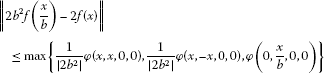(2.13)

for all $x\in \mathcal{A}$. It follows from (2.11), (2.12) and (2.13) that

$\parallel f\left(2x\right)-4f\left(x\right)\parallel \le \psi \left(x\right)$
(2.14)

for all $x\in \mathcal{A}$. For every $g,h:\mathcal{A}\to \mathcal{B}$, define

$d\left(g,h\right):=inf\left\{C\in \left(0,\mathrm{\infty }\right):\parallel g\left(x\right)-h\left(x\right)\parallel \le C\psi \left(x\right),\mathrm{\forall }x\in \mathcal{A}\right\}.$

Hence, d defines a complete generalized non-Archimedean metric on $\mathrm{\Omega }:=\left\{g|g:\mathcal{A}\to \mathcal{B},g\left(0\right)=0\right\}$ (see [27, 28, 33]). Let $T:\mathrm{\Omega }\to \mathrm{\Omega }$ be defined by $Tg\left(x\right)=\frac{1}{{4}^{\ell }}g\left({2}^{\ell }x\right)$ for all $x\in \mathcal{A}$ and all $g\in \mathrm{\Omega }$. If for some $g,h\in \mathrm{\Omega }$ and $C>0$,

$\parallel g\left(x\right)-h\left(x\right)\parallel \le C\psi \left(x\right)$

for all $x\in \mathcal{A}$, then

$\parallel Tg\left(x\right)-Th\left(x\right)\parallel \le \frac{1}{{|4|}^{\ell }}\parallel g\left({2}^{\ell }x\right)-h\left({2}^{\ell }x\right)\parallel \le \frac{C}{{|4|}^{\ell }}\psi \left({2}^{\ell }x\right)\le LC\psi \left(x\right)$

for all $x\in \mathcal{A}$, so

$d\left(Tg,Th\right)\le Ld\left(g,h\right).$

Hence T is a strictly contractive mapping on Ω with the Lipschitz constant L. It follows from (2.14) by using (2.2) that

$\parallel f\left(x\right)-4f\left(\frac{x}{2}\right)\parallel \le \psi \left(\frac{x}{2}\right)\le \frac{L}{|4|}\psi \left(x\right)$

and

$\parallel f\left(x\right)-\frac{1}{4}f\left(2x\right)\parallel \le \frac{1}{|4|}\psi \left(x\right)$

for all $x\in \mathcal{A}$, that is, $d\left(f,Tf\right)\le \frac{{L}^{\frac{1-\ell }{2}}}{|4|}<\mathrm{\infty }$.

Now, by the non-Archimedean alternative contraction principle, T has a unique fixed point $\mathcal{H}:\mathcal{A}\to \mathcal{B}$ in the set $\mathrm{\Lambda }=\left\{g\in \mathrm{\Omega }:d\left(f,g\right)<\mathrm{\infty }\right\}$, which $\mathcal{H}$ is defined by

$\mathcal{H}\left(x\right)=\underset{n\to \mathrm{\infty }}{lim}{T}^{n}f\left(x\right)=\underset{n\to \mathrm{\infty }}{lim}\frac{1}{{4}^{\ell n}}f\left({2}^{\ell n}x\right)$
(2.15)

for all $x\in \mathcal{A}$. By (2.2),

$\underset{n\to \mathrm{\infty }}{lim}\frac{1}{{|4|}^{\ell \left(\ell +2\right)n}}\phi \left({2}^{\ell n}x,{2}^{\ell n}y,{2}^{\ell n}z,{2}^{\ell n}w\right)=0$
(2.16)

for all $x,y,z,w\in \mathcal{A}$. It follows from (2.1), (2.15) and (2.16) that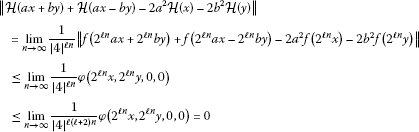for all $x,y\in \mathcal{A}$. This shows that $\mathcal{H}$ is quadratic. Also,

$\begin{array}{rcl}\parallel \mathcal{H}\left(zw\right)-\mathcal{H}\left(z\right)\mathcal{H}\left(w\right)\parallel & =& \underset{n\to \mathrm{\infty }}{lim}\frac{1}{{|4|}^{2\ell n}}\parallel f\left({4}^{\ell n}zw\right)-f\left({2}^{\ell n}z\right)f\left({2}^{\ell n}w\right)\parallel \\ \le & \underset{n\to \mathrm{\infty }}{lim}\frac{1}{{|4|}^{2\ell n}}\phi \left(0,0,{2}^{\ell n}z,{2}^{\ell n}w\right)\\ \le & \underset{n\to \mathrm{\infty }}{lim}\frac{1}{{|4|}^{\ell \left(\ell +2\right)n}}\phi \left(0,0,{2}^{\ell n}z,{2}^{\ell n}w\right)=0\end{array}$

for all $z,w\in \mathcal{A}$. Therefore, $\mathcal{H}$ is a quadratic homomorphism. Moreover, by the non-Archimedean alternative contraction principle,

$d\left(f,\mathcal{H}\right)\le d\left(f,Tf\right)\le \frac{{L}^{\frac{1-\ell }{2}}}{|4|}.$

This implies the inequality (2.3) holds. □

Corollary 2.2 Let $\mathcal{A}$, $\mathcal{B}$ be non-Archimedean Banach algebras over ${\mathbb{Q}}_{2}$, $\ell \in \left\{-1,1\right\}$ be fixed and θ, r be non-negative real numbers such that $\ell r>2\ell \left(\ell +2\right)$. Suppose that a mapping $f:\mathcal{A}\to \mathcal{B}$ satisfies $f\left(0\right)=0$ and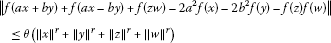for all $x,y,z,w\in \mathcal{A}$, where a, b are positive fixed integers. Then there exists a unique quadratic homomorphism $H:\mathcal{A}\to \mathcal{B}$ such that

$\parallel f\left(x\right)-\mathcal{H}\left(x\right)\parallel \le {2}^{\frac{\ell \left(4-r\right)+r}{2}}\theta {\parallel x\parallel }^{r}\left\{\begin{array}{cc}4,\hfill & gcd\left(a,2\right)=gcd\left(b,2\right)=1;\hfill \\ max\left\{1+{2}^{ir},4\right\},\hfill & a=k{2}^{i},gcd\left(b,2\right)=1;\hfill \\ max\left\{1+{2}^{jr},{2}^{2j+2}\right\},\hfill & gcd\left(a,2\right)=1,b=m{2}^{j};\hfill \\ max\left\{{2}^{ir}+{2}^{jr},{2}^{2j+2}\right\},\hfill & a=k{2}^{i},b=m{2}^{j};\hfill \end{array}$

for all $x\in \mathcal{A}$, where $i,j,k,m\ge 1$ are integers and $gcd\left(k,2\right)=gcd\left(m,2\right)=1$.

Proof The proof follows from Theorem 2.1, by taking

$\phi \left(x,y,z,w\right)=\theta \left({\parallel x\parallel }^{r}+{\parallel y\parallel }^{r}+{\parallel z\parallel }^{r}+{\parallel w\parallel }^{r}\right)$

for all $x,y,z,w\in \mathcal{A}$. Then we choose $L={2}^{\ell \left(2\ell +4-r\right)}$ and we get the desired result. □

Corollary 2.3 Let $\mathcal{A}$, $\mathcal{B}$ be non-Archimedean Banach algebras over ${\mathbb{Q}}_{2}$, $\ell \in \left\{-1,1\right\}$ be fixed and δ, s be non-negative real numbers such that $\ell s>2\ell \left(\ell +2\right)$. Suppose that a mapping $f:\mathcal{A}\to \mathcal{B}$ satisfies $f\left(0\right)=0$ andfor all $x,y,z,w\in \mathcal{A}$, where a, b are positive fixed integers. Then there exists a unique quadratic homomorphism $H:\mathcal{A}\to \mathcal{B}$ such that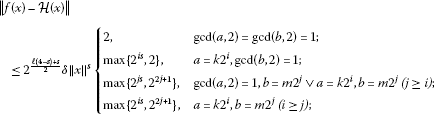for all $x\in \mathcal{A}$, where $i,j,k,m\ge 1$ are integers and $gcd\left(k,2\right)=gcd\left(m,2\right)=1$.

Theorem 2.4 Let $\ell \in \left\{-1,1\right\}$ be fixed and let $f:\mathcal{A}\to \mathcal{A}$ be a mapping with $f\left(0\right)=0$ if $\ell =-1$, for which there exists a function $\phi :{\mathcal{A}}^{4}\to \left[0,\mathrm{\infty }\right)$ satisfying (2.2) and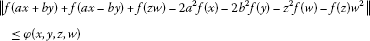(2.17)

for all $x,y,z,w\in \mathcal{A}$ and nonzero fixed integers a, b. Then there exists a unique quadratic derivation $\mathcal{D}:\mathcal{A}\to \mathcal{A}$ such that

$\parallel f\left(x\right)-\mathcal{D}\left(x\right)\parallel \le \frac{{L}^{\frac{1-\ell }{2}}}{|4|}\psi \left(x\right)$
(2.18)

for all $x\in \mathcal{A}$, where $\psi \left(x\right)$ is defined as in Theorem 2.1.

Proof By (2.2), if $\ell =1$, we obtain $\phi \left(0,0,0,0\right)=0$. Letting $x=y=z=w=0$ in (2.17), we get $f\left(0\right)\le \phi \left(0,0,0,0\right)$. So $f\left(0\right)=0$ for $\ell =1$.

By the same reasoning as that in the proof of Theorem 2.1, there exists a unique quadratic mapping $\mathcal{D}:\mathcal{A}\to \mathcal{A}$ satisfying (2.18). The mapping $\mathcal{D}:\mathcal{A}\to \mathcal{A}$ is given by $\mathcal{D}\left(x\right)={lim}_{n\to \mathrm{\infty }}{T}^{n}f\left(x\right)={lim}_{n\to \mathrm{\infty }}\frac{1}{{4}^{\ell n}}f\left({2}^{\ell n}x\right)$ for all $x\in \mathcal{A}$. It follows from (2.17) that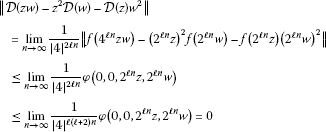for all $z,w\in \mathcal{A}$. Therefore $\mathcal{D}:\mathcal{A}\to \mathcal{A}$ is a quadratic derivation satisfying (2.18). □

Very recently, J. M. Rassias  considered the Cauchy difference controlled by the product and sum of powers of norms, that is, $\theta \left\{{\parallel x\parallel }^{p}{\parallel y\parallel }^{p}+\left({\parallel x\parallel }^{2p}+{\parallel y\parallel }^{2p}\right)\right\}$.

Corollary 2.5 Let $\mathcal{A}$ be a non-Archimedean Banach algebra over ${\mathbb{Q}}_{2}$, $\ell \in \left\{-1,1\right\}$ be fixed and ε, p, q be non-negative real numbers such that $\ell \left(p+q\right)>2\ell \left(\ell +2\right)$. Suppose that a mapping $f:\mathcal{A}\to \mathcal{A}$ satisfies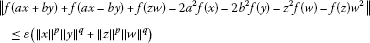for all $x,y,z,w\in \mathcal{A}$, where a, b are positive fixed integers. Then there exists a unique quadratic derivation $\mathcal{D}:\mathcal{A}\to \mathcal{A}$ such that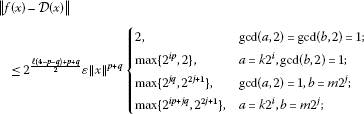for all $x\in \mathcal{A}$, where $i,j,k,m\ge 1$ are integers and $gcd\left(k,2\right)=gcd\left(m,2\right)=1$.

## References

1. Pólya G, Szegő G I. In Aufgaben und Lehrsätze aus der Analysis. Springer, Berlin; 1925.

2. Ulam SM: A Collection of Mathematical Problems. Interscience, New York; 1960.

3. Hyers DH: On the stability of the linear functional equation. Proc. Natl. Acad. Sci. USA 1941, 27: 222–224. 10.1073/pnas.27.4.222

4. Aoki T: On the stability of the linear transformation in Banach spaces. J. Math. Soc. Jpn. 1950, 2: 64–66. 10.2969/jmsj/00210064

5. Rassias TM: On the stability of the linear mapping in Banach spaces. Proc. Am. Math. Soc. 1978, 72: 297–300. 10.1090/S0002-9939-1978-0507327-1

6. Gǎvruta P: A generalization of the Hyers-Ulam-Rassias stability of approximately additive mappings. J. Math. Anal. Appl. 1994, 184: 431–436. 10.1006/jmaa.1994.1211

7. Rassias JM: On approximation of approximately linear mappings by linear mappings. J. Funct. Anal. 1982, 46: 126–130. 10.1016/0022-1236(82)90048-9

8. Rassias JM: Solution of a problem of Ulam. J. Approx. Theory 1989, 57(3):268–273. 10.1016/0021-9045(89)90041-5

9. Rassias JM: On the stability of the Euler-Lagrange functional equation. Chin. J. Math. 1992, 20(2):185–190.

10. Rassias JM: Complete solution of the multi-dimensional problem of Ulam. Discuss. Math. 1994, 14: 101–107.

11. Gǎvruta P: An answer to a question of John M. Rassias concerning the stability of Cauchy equation. Hardronic Math. Ser. Advances in Equations and Inequalities 1999, 67–71.

12. Bourgin DG: Approximately isometric and multiplicative transformations on continuous function rings. Duke Math. J. 1949, 16: 385–397. 10.1215/S0012-7094-49-01639-7

13. Badora R: On approximate ring homomorphisms. J. Math. Anal. Appl. 2002, 276: 589–597. 10.1016/S0022-247X(02)00293-7

14. Šemrl P: The functional equation of multiplicative derivation is superstable on standard operator algebras. Integral Equ. Oper. Theory 1994, 18: 118–122. 10.1007/BF01225216

15. Badora R: On approximate derivations. Math. Inequal. Appl. 2006, 9: 167–173.

16. Arriola LM, Beyer WA: Stability of the Cauchy functional equation over p -adic fields. Real Anal. Exch. 2005/2006, 31: 125–132.

17. Cho YJ, Park C, Saadati R: Functional inequalities in non-Archimedean Banach spaces. Appl. Math. Lett. 2010, 23: 1238–1242. 10.1016/j.aml.2010.06.005

18. Cho YJ, Saadati R: Lattice non-Archimedean random stability of ACQ functional equation. Adv. Differ. Equ. 2011., 2011: Article ID 31

19. Cho YJ, Saadati R, Vahidi J:Approximation of homomorphisms and derivations on non-Archimedean Lie ${C}^{\ast }$-algebras via fixed point method. Discrete Dyn. Nat. Soc. 2012., 2012: Article ID 373904

20. Eshaghi Gordji M, Khodabakhsh R, Khodaei H, Park C, Shin DY: A functional equation related to inner product spaces in non-Archimedean normed spaces. Adv. Differ. Equ. 2011., 2011: Article ID 37

21. Eshaghi Gordji M, Khodaei H, Khodabakhsh R: General quartic-cubic-quadratic functional equation in non-Archimedean normed spaces. U. P. B. Sci. Bull., Ser. A 2010, 72: 69–84.

22. Eshaghi Gordji M, Savadkouhi MB: Stability of cubic and quartic functional equations in non-Archimedean spaces. Acta Appl. Math. 2010, 110: 1321–1329. 10.1007/s10440-009-9512-7

23. Eshaghi Gordji M, Savadkouhi MB: Stability of a mixed type cubic-quartic functional equation in non-Archimedean spaces. Appl. Math. Lett. 2010, 23: 1198–1202. 10.1016/j.aml.2010.05.011

24. Najati A, Cho YJ: Generalized Hyers-Ulam stability of the pexiderized Cauchy functional equation in non-Archimedean spaces. Fixed Point Theory Appl. 2011., 2011: Article ID 309026

25. Radu V: The fixed point alternative and the stability of functional equations. Fixed Point Theory 2003, 4: 91–96.

26. Diaz JB, Margolis B: A fixed point theorem of the alternative for contractions on the generalized complete metric space. Bull. Am. Math. Soc. 1968, 126: 305–309.

27. Cădariu L, Radu V: Fixed points and the stability of Jensen’s functional equation. J. Inequal. Pure Appl. Math. 2003., 4: Article ID 4

28. Cădariu L, Radu V: On the stability of the Cauchy functional equation: A fixed point approach. Grazer Math. Ber. 2004, 346: 43–52.

29. Cădariu L, Radu V: Fixed point methods for the generalized stability of functional equations in a single variable. Fixed Point Theory Appl. 2008., 2008: Article ID 749392

30. Cho YJ, Kang JI, Saadati R: Fixed points and stability of additive functional equations on the Banach algebras. J. Comput. Anal. Appl. 2012, 14: 1103–1111.

31. Gǎvruta P, Gǎvruta L: A new method for the generalized Hyers-Ulam-Rassias stability. Int. J. Nonlinear Anal. Appl. 2010, 1(2):11–18.

32. Jung S, Kim T: A fixed point approach to the stability of the cubic functional equation. Bol. Soc. Mat. Mexicana 2006, 12: 51–57.

33. Mirmostafaee AK: Hyers-Ulam stability of cubic mappings in non-Archimedean normed spaces. Kyungpook Math. J. 2010, 50: 315–327. 10.5666/KMJ.2010.50.2.315

34. Eshaghi Gordji M, Khodaei H: On the generalized Hyers-Ulam-Rassias stability of quadratic functional equations. Abstr. Appl. Anal. 2009., 2009: Article ID 923476

35. Eshaghi Gordji M: Nearly ring homomorphisms and nearly ring derivations on non-Archimedean Banach algebras. Abstr. Appl. Anal. 2010., 2010: Article ID 393247

36. Hensel K: Uber eine neue Begrundung der Theorie der algebraischen Zahlen. Jahresber. Dtsch. Math.-Ver. 1897, 6: 83–88.

37. Khrennikov A: p-adic Valued Distributions in Mathematical Physics. Kluwer Academic, Dordrecht; 1994.

38. Khrennikov A: Non-Archimedean Analysis: Quantum Paradoxes, Dynamical Systems and Biological Models. Kluwer Academic, Dordrecht; 1997.

39. Gouvêa FQ: p-adic Numbers. Springer, Berlin; 1997.

40. Vladimirov VS, Volovich IV, Zelenov EI: p-adic Analysis and Mathematical Physics. World Scientific, Singapore; 1994.

41. Murphy, GJ: Non-Archimedean Banach algebras. PhD thesis, University of Cambridge (1977)

42. Narici L: Non-Archimedian Banach spaces and algebras. Arch. Math. 1968, 19: 428–435. 10.1007/BF01898426

43. Ravi K, Arunkumar M, Rassias JM: Ulam stability for the orthogonally general Euler-Lagrange type functional equation. Int. J. Math. Stat. 2008, 3: 36–46.

44. Bouikhalene B, Elqorachi E, Rassias JM: The superstability of d’Alembert’s functional equation on the Heisenberg group. Appl. Math. Lett. 2010, 23: 105–109. 10.1016/j.aml.2009.08.013

45. Cao H-X, Lv J-R, Rassias JM: Superstability for generalized module left derivations and generalized module derivations on a Banach module. I. J. Inequal. Appl. 2009., 2009: Article ID 718020

46. Cao H-X, Lv J-R, Rassias JM: Superstability for generalized module left derivations and generalized module derivations on a Banach module. II. J. Inequal. Pure Appl. Math. 2009., 10(3): Article ID 85

## Author information

Authors

### Corresponding authors

Correspondence to Madjid Eshaghi Gordji or Choonkil Park.

### Competing interests

The authors declare that they have no competing interests.

### Authors’ contributions

All authors read and approved the final manuscript.

## Rights and permissions

Reprints and Permissions

Eshaghi Gordji, M., Khodaei, H., Khodabakhsh, R. et al. Fixed points and quadratic equations connected with homomorphisms and derivations on non-Archimedean algebras. Adv Differ Equ 2012, 128 (2012). https://doi.org/10.1186/1687-1847-2012-128

• Accepted:

• Published:

• DOI: https://doi.org/10.1186/1687-1847-2012-128

### Keywords

• fixed point approach
• non-Archimedean algebra